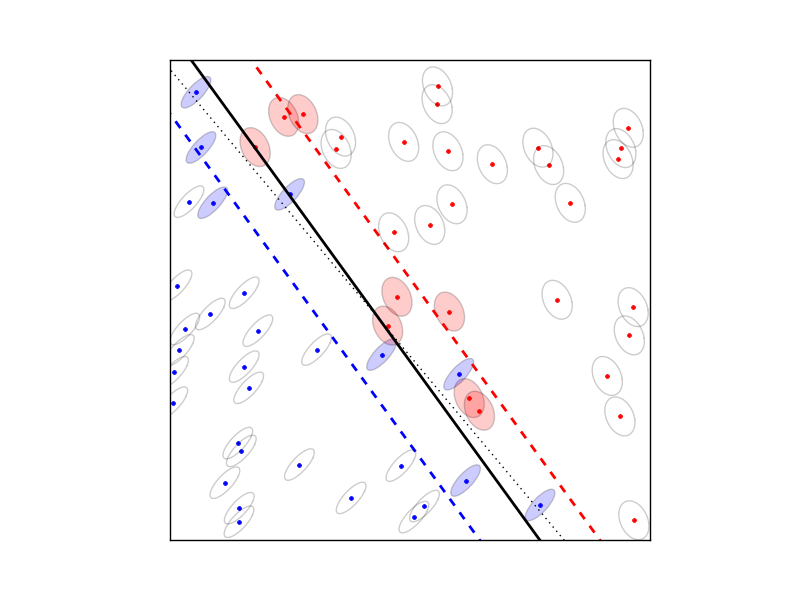# Robust SVM

The robust SVM training problem can be expressed as a cone QP with second-order cone constraints:

$\begin{split}\begin{array}{ll} \mbox{minimize} & (1/2) w^Tw + \gamma \mathbf{1}^Tv \\ \mbox{subject to} & \mathbf{diag}(d)(Xw + b \mathbf{1} ) \succeq 1 - v + Eu \\ & v \succeq 0 \\ & \| S_j w\|_2 \leq u_j, \quad j =1,\ldots,t. \end{array}\end{split}$

The variables are $$w \in \mathbf{R}^n$$, $$b \in \mathbf{R}$$, $$v \in \mathbf{R}^N$$, and $$u \in \mathbf{R}^t$$. The matrix $$X \in \mathbf{R}^{N\times n}$$ has as its rows the training examples $$x_i^T$$ and the vector $$d \in \{-1, 1\}^N$$ contains the training labels. The matrices $$S_j$$ define the shape and the size of the uncertainty ellipsoids, and the matrix $$E$$ is a selector matrix with zeros and one ‘1’ per row. $$E_{ij} = 1$$ means that the i’th training vector is associated with the j’th uncertainty ellipsoid. For $$t = 0$$, the term $$Eu$$ and the norm constraints are absent, and the problem reduces to the standard linear SVM

$\begin{split}\begin{array}{ll} \mbox{minimize} & (1/2) w^Tw + \gamma \mathbf{1}^Tv \\ \mbox{subject to} & \mathbf{diag}(d)(Xw + b \mathbf{1} ) \succeq 1 - v \\ & v \succeq 0. \end{array}\end{split}$

### Documentation

A custom solver for the robust SVM problem is available as a Python module robsvm.py. The module implements the following function:

robsvm(X, labels, gamma, P, e)

Solves the ‘soft-margin’ robust SVM problem.

The first three input arguments are the data matrix $$X$$ (with the $$N$$ training examples $$x_i^T$$ as rows), the label vector $$d$$, and the positive parameter $$\gamma$$. The fourth input argument P must be a Python list of $$t$$ matrices $$S_1,\ldots,S_t$$. The last argument e is an $$N$$-vector where the i’th element $$e_i \in \{0,..,t-1\}$$ is the index of the uncertainty ellipsoid associated with the i’th training vector.

The function returns $$w$$, $$b$$, $$u$$, $$v$$, and the number of iterations (an integer).

### Example

from robsvm import robsvm
from cvxopt import matrix, normal, uniform

# parameters
m, n = 60, 2
gamma = 10.0

# generate random problem data
X = 2.0*uniform(m,n)-1.0
d = matrix(1,(m,1))

# generate noisy labels
w0 = matrix([2.0,1.0])+normal(2,1); b0 = 0.4
z = 0.2*normal(m,1)
for i in range(m):
if (X[i,:]*w0) + b0 < z[i]: d[i] = -1

# generate uncertainty ellipsoids
k = 2
P = [0.1*normal(4*n,n) for i in range(k)]
P = [ p.T*p for p in P]
e = matrix(0,(m,1))
for i in xrange(m):
if d[i] == -1: e[i] = 1

# solve SVM training problem
w, b, u, v, iterations = robsvm(X, d, gamma, P, e)